Mr BM

•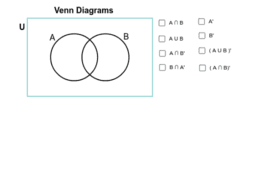Copy of Venn Diagrams

Activity

Mr BM

•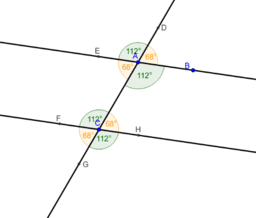Copy of Angles of Parallel Lines

Activity

Mr BM

•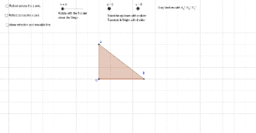Copy of Geometric Transformations

Activity

Mr BM

•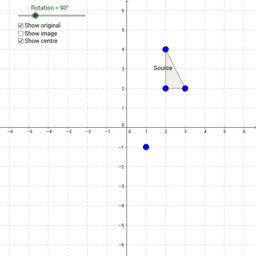Copy of Transformations - Rotation

Activity

Mr BM

•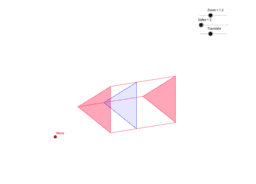Copy of Constant Cross-Sectional Area of a Prism

Activity

Mr BM

•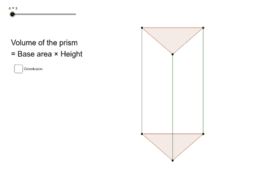Copy of Prisms & Cylinders

Activity

Mr BM

•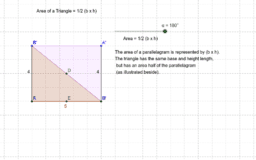Area of a Triangle

Activity

Mr BM

•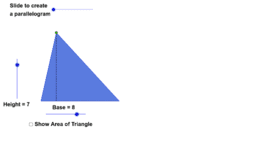Area Of A Triangle

Activity

Mr BM

•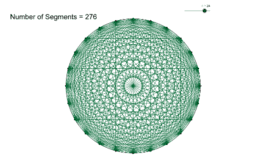Mystic Rose and Handshakes

Activity

Mr BM

•Surface Area for Cuboid

Activity

Mr BM

•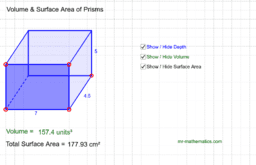Prisms Volume and Surface Area

Activity

Mr BM

•Equivalents fractions

Activity

Mr BM

•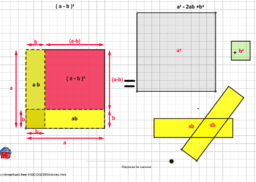(a - b)²

Activity

Mr BM

•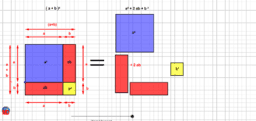(a+b)²

Activity

Mr BM

•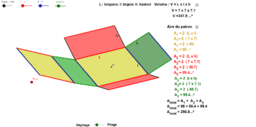Volume and lateral area of a box

Activity

Mr BM

•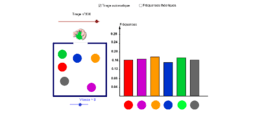Probability in a box

Activity

Mr BM

•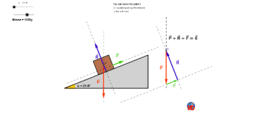Inclined plane

Activity

Mr BM

•Polar boat game (update in version 4)

Activity

Mr BM

•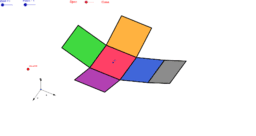The 11 paterns of the cube ? (Net of a Cube)

Activity

Mr BM

•The 11 paterns of the cube ? (Net of a Cube)

Activity

Mr BM

•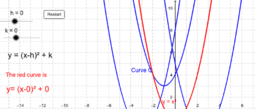Quadratic curves and Equations Set 6 h&k

Activity

Mr BM

•The Circle Theorem

Activity

Mr BM

•Equations of Parallel Lines

Activity

Mr BM

•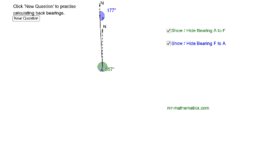Activity

Mr BM